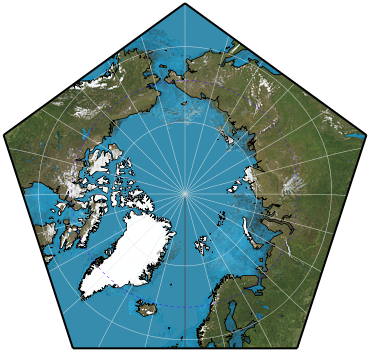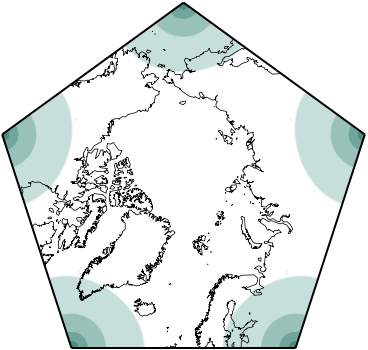## Geocart Projections

What is a projection?

Previous | Next

polyhedron conformal faceParameters: Platonic solid, Face

Description

World projected conformally into any face of any platonic polyhedron. There are five platonic solids, each consisting of the same shape repeated some numbers of times, and each foldable into an approximation of a sphere:
1. Tetrahedron (four faces, each a triangle)
2. Cube (six faces, each a square)
3. Octahedron (eight faces, each a triangle)
4. Dodecahedron (twelve faces, each a pentagon)
5. Icosahedron (twenty faces, each a triangle)

Classifications

Conformal
Polyhedral

Graticule

Meridians: Complex curves except for the edges and central meridian of each facet
Parallels: Complex curves except for straight edge at base of each facet

Distortion

No distortion at the centroid of each face. Maximum distortion at the corners.

Parameters

Platonic solid: choice of “tetrahedron”, “cube”, “octahedron”, “dodecahedron”, “icosahedron”.
Face: An integer from 0 through n-1, where n is the number of faces in the polyhedron.

Origin

Mathematical work on conformal projections for regular polyhedra largely originated with Oscar S. Adams from 1925 and onward. L.P. Lee greatly expanded upon and regularized the mathematics in 1976.